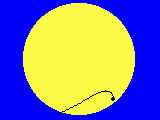Essen, horizontal view

# Transit of Venus, June 8th, 2004Essen, equatorial view

## Project 2: Determing of the own geographical coordinates and the projected distance of different observers

To be able to derive an own measure of the Astronomical Unit from the self determined value of the Sun's parallax by the following equation (see the related paper (in German or in English))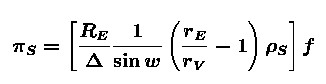you need firstly to determine, among other quantities, the distance Δ between the two observers or, to be more precise, the relation Δ/RE between the distance and the radius of the Earth. Secondly, you must determine the angle of projection w because you must calculate the the projection of the distance parallel to the direction to the Sun.

The distance Δ can be calculated when the geographical coordinates of the both observers are known. For the calculation of w it is necessary to determine the local sideral time of the observers and the position of the Sun, that means its geocentric equatorial coordinates.

There are two tasks for measurements:

1. Determination of the own geographical position. This may be done in advance.
2. Measurement of the Sun's position on June 8th which can be done best at noon.
Of course, very exact measures of both quantities are easily to be obtain, the geographical position, for instance, by GPS (Global Positioning System), the position of the Sun from every astronomical almanach or computer program. Nevertheless, we will try to get own measures in order to understand better the foundations of the astronomical distance ladder!

Because the geographical positions influence the result for the parallax of the Sun only weakly quite rough methods of "astronomical navigation" are sufficient.

## 1. The geographical position

For these measurements, it is suitable to have determined the exact direction to south in advance (project 3)!

### 1.1 Geographical latitude

#### 1.1.1 at night

The geographical latitude equals the elevation of the northern celestial pole and, with sufficient accuracy, that of Polar Star: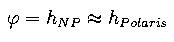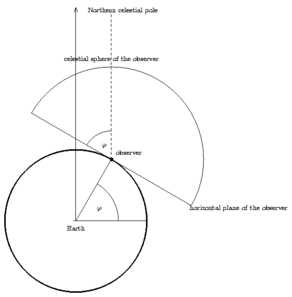Unfortunately, there is no as simple method for observers on the southern hemisphere! But it is possible to apply the method which is described for the Sun, below, to a star with known declination.

#### 1.1.2 during the daytime

The simplest method is to measure the elevation of the Sun at noon. The best way to do that is described in project 3 where not the maximal elevation but the time of culmination is of interest.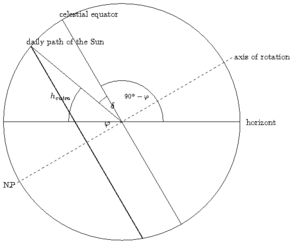maximal elevation for northern sites maximal elevation for southern sites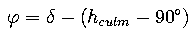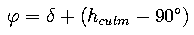### 1.2 Geographical longitude

Measurements of longitude are measurements of time! (A possible device is pictured in project 4.)

#### 1.2.1 at night

If you know the local sideral time you can derive the longitude by the following algorithm:

• Your actual sideral time differ from that of Greenwich by 4min/degree times your longitude (which we count positive if your site is east of Greenwich):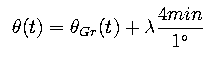• The sideral time at the time t(UT) can be derived from that at midnight:The factor 1.0027379 is due to the fact that, during 24 hours, the Earth rotates more than once and, therefore, more than 24 h sideral time pass.
• The Greenwich sideral time at midnight of the day of interest may be taken from an astronomical almanach. Combining these relations we find the following equation which makes it possible to derive the longitude from the local sideral time at t: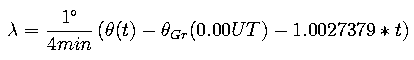The simplest way to determine local sideral time is to use the "celestial clock":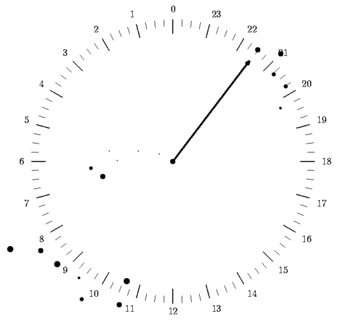A more precise way is to measure the time of culmination of a star with known right ascension: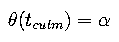To be able to recognize the time of culmination you should know the exact direction to south.

#### 1.2.2 during the daytime

In this case, you should measure the time of local midday, that is the time at which the Sun culminates, by the method described in project 3: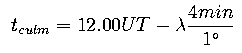If you live east of Greenwich the Sun will culminate before 12.00 UT! For exactness, this equation had to be corrected by the equation of time. But because in June this correction would be small it has been omitted.

Your longitude, therefore, can be calculated in the following way: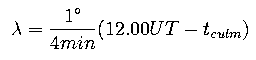## 2. The position of the Sun

The position of the Sun ca be determined by the same procedures as described above (Caution: There is a certain danger to get into a tautology!)
1. The declination can be determined by measuring the maximal elevation.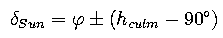2. The right ascension can be determined by observing the exact time of culmination and calculating the associative sideral time.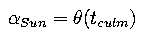## 3. The angle of projection

To be able to calculate the angle of projection w you must know the vector connecting two observers and the vector directing to the Sun in the same coordinate system. As we already know the position of the Sun in geocentric equatorial coordinates we want to transform the positions of the observers in that system, too.• As the picture above shows the declination of an observers equals, obviously, his geographical latitude;• When the observer is located in the x-y-plane (as in the picture above) his right ascension is, obviously, zero. Additionally, the vernal equinox is just culminating for him, that is his local sideral time is 0.00h. Then, due to the rotation of the Earth his right ascension is growing with the same rate as his sideral time. Therefore, his right ascension always equals his sideral time: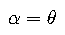Pooh, a lot of work have been done. But now it is easy, at least in principle, to calculate the angle of projection:

1. Transform the equatorial positions of the observers from polar into rectangular coordinates: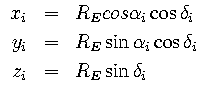The rectangular positions are then given by: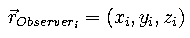2. The distance, therefore, is the length of the vector connecting the both observers: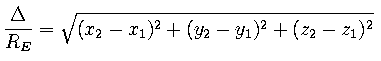3. Transform, in the same manner, the position of the Sun to rectangular coordinates.

4. The angle of projection can then be calculated with the scalar product:Finally, we get the projection angle from the following equation: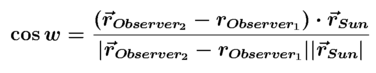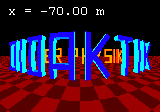Prof. Dr. Udo Backhaus
last modification:  March 28th, 2008